# SSC Reasoning Ability Practice Questions (Day-78)

Dear Aspirants, The SSC Exams are going on at national level. Millions of candidates have eagerly prepared for the ongoing SSC exams such as CGL, CHSL, MTS, CPO, JE etc. SSC exams are usually conducted by the Staff Selection Commission to recruit the eligible candidates for various Central government departments. Mostly the selection process for all the SSC exams consists of the single or double phase of written examination based on the examination. Due to that, the written exam consisted of a certain syllabus and exam pattern. By following those regulations, the written exam has been conducted through an online mode. Based on the latest updated exam pattern and syllabus, here we have drafted the Reasoning Ability questions on a daily basis. So aspirants start your practice session with us and mould your skill in a perfect shape.

Start Quiz

Direction (1-2): Which answer figure will complete the pattern in the question figure?

1)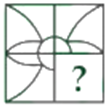(a)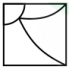(b)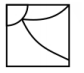(c)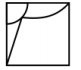(d)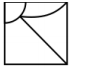2)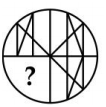(a)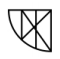(b)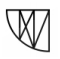(c)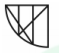(d)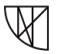Direction (3-4): From the given answer figures, select the one in which the question figure is hidden/embedded.

3)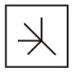(a)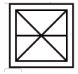(b)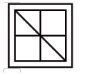(c)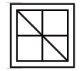(d)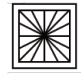4)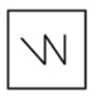(a)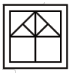(b)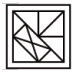(c)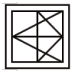(d)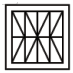Direction (5-6): A piece of paper is folded and punched as shown below in the question figures. From the given answer figures, indicate how it will appear when opened?

5)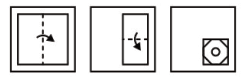(a)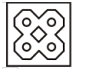(b)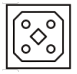(c)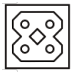(d)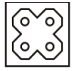6)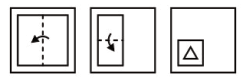(a)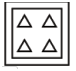(b)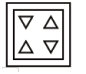(c)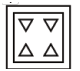(d)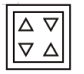Direction (7-8): If a mirror is placed on the line AB, then which of the answer figures is the right image of the given figure?

7)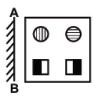(a)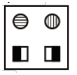(b)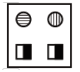(c)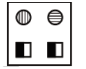(d)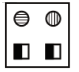8)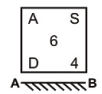(a)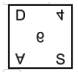(b)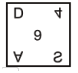(c)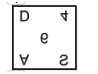(d)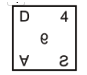9) A word is represented by only one set of numbers as given in any one of the alternatives. The sets of numbers given in the alternatives are represented by two classes of alphabets as shown in the given two matrices. The columns and rows of Matrix-I are numbered from 0 to 4 and that of Matrix-II are numbered from 5 to 9. A letter from these matrices can be represented first by its row and next by its column, for example, ‘E’ can be represented by 10, 22, etc, and ‘O’ can be represented by 55, 98, etc. Similarly, you have to identify the set for the word “
PROBE”.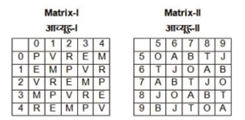(a) 00, 33, 86, 95, 04

(b) 43, 40, 55, 88, 34

(c) 31, 02, 67, 56, 04

(d) 24, 21,12, 96, 00

10) A word is represented by only one set of numbers as given in any one of the alternatives. The sets of numbers given in the alternatives are represented by two classes of alphabets as shown in the given two matrices. The columns and rows of Matrix-I are numbered from 0 to 4 and that of Matrix-II are numbered from 5 to 9. A letter from these matrices can be represented first by its row and next by its column, for example, ‘V’ can be represented by 20, 44, etc., and ‘R’ can be represented by 66, 97, etc., Similarly, you have to identify the set for the word “SLAIN”.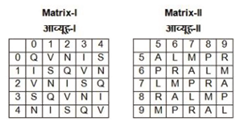(a) 04, 68, 55, 23, 20

(b) 30, 55, 56, 03, 32

(c) 23, 56, 67, 34, 40

(d) 11, 68, 66, 14, 21

Answers :

1) Answer: A

2) Answer: D

3) Answer: D

4) Answer: D

5) Answer: A

6) Answer: C

7) Answer: A

8) Answer: C

9) Answer: B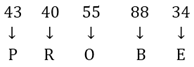10) Answer: C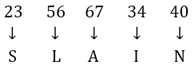Check Here to View SSC CGL / CHSL 2021 Reasoning Ability Questions Day – 77 Day – 76 Day – 75
3 1 vote
Rating0 Comments
Inline Feedbacks
View all comments